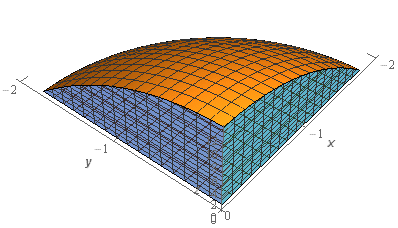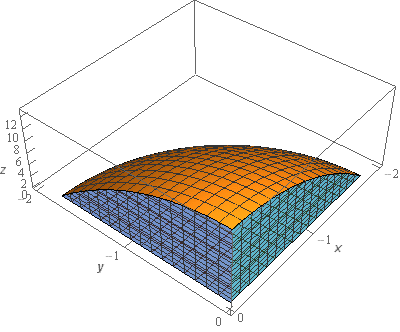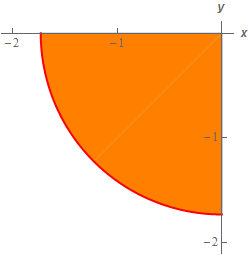Paul's Online Notes
Home / Calculus III / Multiple Integrals / Surface Area
Show Mobile Notice Show All Notes Hide All Notes
Mobile Notice
You appear to be on a device with a "narrow" screen width (i.e. you are probably on a mobile phone). Due to the nature of the mathematics on this site it is best views in landscape mode. If your device is not in landscape mode many of the equations will run off the side of your device (should be able to scroll to see them) and some of the menu items will be cut off due to the narrow screen width.

### Section 15.9 : Surface Area

2. Determine the surface area of the portion of $$z = 13 - 4{x^2} - 4{y^2}$$ that is above $$z = 1$$ with $$x \le 0$$ and $$y \le 0$$.

Show All Steps Hide All Steps

Start Solution

Okay, let’s start off with a quick sketch of the surface so we can get a feel for what we’re dealing with.We’ve given the sketches with a set of “traditional” axes as well as a set of “box” axes to help visualize the surface.

The surface we are after is the portion of the elliptic paraboloid (the orange surface in the sketch) that is above $$z = 1$$ and in the 3rd quadrant of the $$xy$$-plane. The bluish “walls” are simply there to provide a frame of reference to help visualize the surface and are not actually part of the surface we are interested in.

Show Step 2

The integral for the surface area is,

$S = \iint\limits_{D}{{\sqrt {{{\left[ { - 8x} \right]}^2} + {{\left[ { - 8y} \right]}^2} + 1} \,dA}} = \iint\limits_{D}{{\sqrt {64{x^2} + 64{y^2} + 1} \,dA}}$ Show Step 3

Now, as implied in the last step $$D$$ must be in the $$xy$$-plane and it should be (hopefully) pretty obvious that it will be a circular region. If you look at the surface from directly above you will see the following region.To determine equation of the circle all we need to do is set the equation of the elliptic paraboloid equal to $$z = 1$$ to get,

$1 = 13 - 4{x^2} - 4{y^2}\hspace{0.25in} \to \hspace{0.25in}4{x^2} + 4{y^2} = 12\hspace{0.25in} \to \hspace{0.25in}{x^2} + {y^2} = 3$

So, it is the portion of the circle of radius $$\sqrt 3$$ in the 3rd quadrant.

At this point it should also be clear that we’ll need to evaluate the integral in terms of polar coordinates. In terms of polar coordinates the limits for $$D$$ are,

$\begin{array} \displaystyle \pi \le \theta \le \frac{{3\pi }}{2}\\ 0 \le r \le \sqrt 3 \end{array}$ Show Step 4

Now, let’s convert the integral into polar coordinates.

$S = \iint\limits_{D}{{\sqrt {64{x^2} + 64{y^2} + 1} \,dA}} = \int_{\pi }^{{\frac{3}{2}\pi }}{{\int_{0}^{{\sqrt 3 }}{{r\sqrt {64{r^2} + 1} \,dr}}\,d\theta }}$

Don’t forget to pick up the extra $$r$$ from converting the $$dA$$ into polar coordinates. If you need a refresher on converting integrals to polar coordinates then you should go back and work some problems from that section.

Show Step 5

Okay, all we need to do then is evaluate the integral.

\begin{align*}S & = \int_{\pi }^{{\frac{3}{2}\pi }}{{\int_{0}^{{\sqrt 3 }}{{r\sqrt {64{r^2} + 1} \,dr}}\,d\theta }}\\ & = \int_{\pi }^{{\frac{3}{2}\pi }}{{\left. {\frac{1}{{192}}{{\left( {64{r^2} + 1} \right)}^{\frac{3}{2}}}} \right|_0^{\sqrt 3 }\,d\theta }}\\ & = \int_{\pi }^{{\frac{3}{2}\pi }}{{\frac{1}{{192}}\left( {{{193}^{\frac{3}{2}}} - 1} \right)\,d\theta }}\\ & = \left. {\frac{1}{{192}}\left( {{{193}^{\frac{3}{2}}} - 1} \right)\theta } \right|_\pi ^{\frac{3}{2}\pi } = \require{bbox} \bbox[2pt,border:1px solid black]{{\frac{\pi }{{384}}\left( {{{193}^{\frac{3}{2}}} - 1} \right) = 21.9277}}\end{align*}